RS Aggarwal Class 10 Solutions Chapter 14 Height And Distance

RS Aggarwal Class 10 Chapter 14 Height And Distance Solutions Free PDF

Heights and distances is a topic closely associated with the topic of Trigonometry and it has many different real-life applications such as Astronomy, Engineering, and Architecture as well. Some of the major topics covered in this chapter are the distance of the object from the observer, height of the object, angle of an object from the observer and the perspective of an object from the observer’s point of view. Students can make different calculations with the help of the Pythagoras theorem, which is primarily used as a method for calculating the different heights and distances.

Check out the RS Aggarwal Class 10 Solutions Chapter 14 Heights and Distances below:

Q.1:  A tower stands vertically on the ground. From a point on the ground which is 20m away from the foot of the tower, the angle of elevation of its top is found to be $60^{\circ}$. Find the height of the tower. [Take $\sqrt{3}=1.732$]

Sol:

Let AB be the tower standing on a level ground and 0 be the position of the observer. Then OA = 20 m and ∠OAB = 90° and ∠AOB = 60°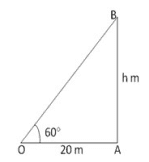Let AB = h meters

From the right ⧍OAB, we have

$\frac{AB}{OA}$ = Tan 60o = √3

$\frac{h}{20}$ = √3

h = (20 x √3)

h = 20 x 1.732

h = 34.64m

Hence the height of the tower is 20√3 m = 34.64m

Q.2: A kite is flying at a height of 75m from the level ground, attached to a string inclined at $60^{\circ}$ to the horizontal. Find the length of the string, assuming that there is no slack in it. [Take $\sqrt{3}=1.732$]

Sol:

Let OB be the length of the string from the level of ground and 0 be the point of the observer, then,

AB = 75m and ∠OAB = 90° and ∠AOB = 60°, let OB = I meters.

From the right ⧍OAB: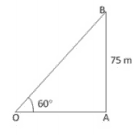$\frac{OB}{AB}$ = cosec 60° = $\frac{2}{√3}$

$\frac{I}{75}$ = $\frac{2}{√3}$

I = ( 75 x $\frac{2}{√3}$ x $\frac{√3}{√3}$ )

I = 25 x 2 x √3

I = 50√3

I = 86.6m

Hence, the length of the string 86.6m

Q.3: The angle of depression from the top of a tower of a point A on the ground is $30^{\circ}$. On moving a distance of 20 meters from the point A towards the foot of the tower to a point B, the angle of elevation of the top of the tower from the point B is $60^{\circ}$. Find the height of the tower and its distance from the point A.

Sol:

Let CD be the tower and BD be the ground

Then, ∠CBD = 30°, ∠CAD = 60°

∠BDC = 90°, AB = 20 m, CD = h metre and AD = x metre.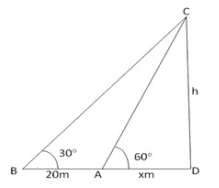From ∆BCD:

$\frac{CD}{BD}$ = tan 30°

= $\frac{CD}{BD}$

$\frac{h}{20 + x}$ = $\frac{1}{√3}$ = √3h = 20 + x

√3h = 20 + x

=> x = √3h – 20.…………(1)

$\frac{CD}{AD}$ = tan 60°

$\frac{h}{x}$ = √3

$\frac{h}{√3}$ = x  ……….(2)

From (1) and (2) we get,

√3h – 20 = $\frac{h}{√3}$

=> 3h – 20√3 = h

=> h = 10√3 = 10×1.732 => 17.32

H = 17.32m and   BD = 30m

Hence, the height of the tower = 17.32m and the distance of the tower from the point A = 30m.

Q.4: A vertical tower stands on a horizontal plane and is surmounted by a vertical flagstaff of height 5 m. At a point on the plane, the angle of elevation of the bottom of the flagstaff is $30^{\circ}$ and that of the top of the flagstaff is $60^{\circ}$. Find the height of the tower. [Use $\sqrt{3}= 1.73$]

Sol:

Let AB be the tower h meter high. CA is the flagstaff 5 meter high.

Let PB = x meter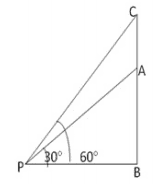In ∆ PBC,

∠CPB = 60°, ∠PBC = 90°

$\frac{BC}{PB}$ = tan 60°

$\frac{5+x}{x}$ = √3

5 + h = √3x  ……….(1)

∠APB = 30° and ∠ABP = 90°

$\frac{AB}{PB}$ = tan 30°

$\frac{h}{x}$ = $\frac{1}{√3}$

√3h = x

Putting value of x in (1), we get

5 + h = √3 x √3h => 3h

2h = 5

Or h = 5/2m

Thus, height of tower = 2.5m

Q.5: A statue 1.46m tall stands on the top of a pedestal. From a point on the ground, the angle of elevation of the top of the statue is $60^{\circ}$ and from the same point, the angle of elevation of the top of the pedestal is $45^{\circ}$. Find the height of the pedestal. [Use $\sqrt{3}= 1.73$]

Sol:

Let SP be the statue and PB be the pedestal. Angles of elevation of S and P are 60° and 45° respectively.

Further suppose AB = x m, PB = h m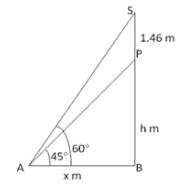In right ∆ ABS,

$\frac{SB}{AB}$ = tan 60° = √3

$\frac{h+1.46}{x}$ = √3  ……(1)

In right  ∆ PAB,

$\frac{PB}{AB}$ = tan 45° = 1

h = x ……….(2)

putting x = h in (1)

$\frac{h + 1.46}{h}$ = √3

=> h + 1.46 = √3 h

h = 0.73 x 2.732 = 2m (nearly)

Thus, the height of the pedestal = 2m.

Q.6: The angle of elevation of the top of a tower from a point on the same level as the foot of the tower is $30^{\circ}$. On advancing 150m towards the foot of the tower, the angle of elevation becomes $60^{\circ}$. Show that the height of the tower is 129.9 meters. [Given $\sqrt{3}=1.732$]

Sol:

Let AB be the tower and let the angle of elevation of its top at C be 30°. Let D be a point at a distance 150m from C such that the angle of elevation of the top of the tower at D is 60°. Let h m be the height of the tower and AD = x m.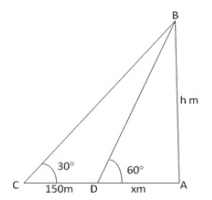In ∆ CAB, we have

Tan 30° = $\frac{AB}{AC}$

=> $\frac{1}{√3}$ = $\frac{h}{x+150}$  ……….(1)

In ∆ DAB, we have

Tan 60° = $\frac{AB}{AD}$ => √3 = $\frac{h}{x}$

X = $\frac{h}{√3}$  ……(2)

Putting the x = $\frac{h}{√3}$   in (1), we get

=> $\frac{1}{√3}$  = $\frac{√3h}{h+150√3}$

=> h+150√3  =  3h  => 3h – h = 150√3

2h = 150√3

h = 75√3

h = (75×1.732)m

h = 129.9

Hence the height of tower is 129.9 m

Q.7: On a horizontal plane there is a vertical tower with a flagpole on the top of the tower. At a point, 9 meters away from the foot of the tower, the angle of elevation of the top and bottom of the flagpole are $60^{\circ}\:and\:30^{\circ}$ respectively. Find the height of the tower and the flagpole mounted on it. [Take $\sqrt{3}=1.73$]

Sol:

Let AB be the tower and BC be the flagpole, Let O be the point of observation. Then, OA = 9 m, ∠AOB = 30° and ∠AOC = 60°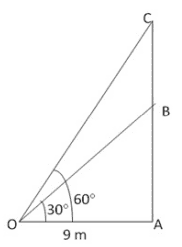From right angled ∆BOA:

$\frac{AB}{OA}$   = tan 30°

$\frac{AB}{9}$ = $\frac{1}{√3}$   => AB = 3√3

From right angled ∆OAC

$\frac{AC}{OA}$   = tan 60°

BC = (AC – AB) => 6√3m.

Thus, AB = 3√3m => 5.196m

BC = 6√3m => 10.392m

Hence, height of the tower= 5.196 m and

height of the flagpole = 10.392 m

Access CBSE Sample Paper for class 10 Maths here.

Access NCERT Book for class 10 Maths here.

Practise This Question

Statement 1: When white light ray passes through a prism it disperses into seven different colours, and this phenomenon is called dispersion.

Statement 2: Refraction depends upon the wavelength of light and since different colours have different wavelengths hence they are refracted at different angle and so they are split into the colour spectrum.